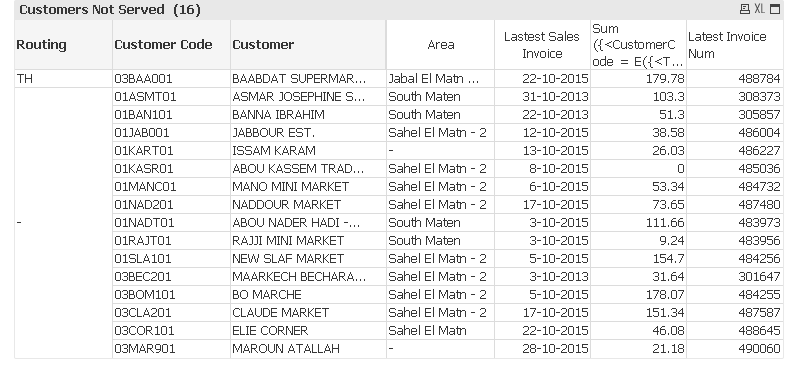# QlikView App Dev

Discussion Board for collaboration related to QlikView App Development.

Announcements
Support Case Portal has moved to Qlik Community! Read the FAQs to start exploring Support resources.
cancel
Showing results for
Did you mean:Partner

## Help in expression

Hello got a pivot table in which I'm getting the latest invoice number and latest invoice date for customers not served in the selected month and year (check attached)

I couldn't get the sum of amount where InvoiceNum is the one gotten in the expression labeled Latest Invoice Num

I can walk on water when it freezes
1 Solution

Accepted SolutionsMVP

May be this:

Sum({<CustomerCode = E({<TrxType={'Sales'},CustomerInactive={'n'},CusNum = {"=Sum({<TrxType={'Sales'}>} ValueUSD)>0"}>} CustomerCode)

,TrxType={'sales'}

,CustomerInactive={'n'}

,YEAR=,MONTH=,MONTH_NUM=,MONTH_YEAR=,DAY=

,TrxDate={'<=\$(vEOM_LastMonth)'}>}

Aggr(If(Only({1}InvoiceNum) = Max(TOTAL <Customer_Default_Salesman, Routing, CusName, CustomerCode> {<CustomerCode = E({<TrxType={'Sales'},CustomerInactive={'n'},CusNum = {"=Sum({<TrxType={'Sales'}>} ValueUSD)>0"}>} CustomerCode)

,TrxType={'sales'}

,CustomerInactive={'n'}

,YEAR=,MONTH=,MONTH_NUM=,MONTH_YEAR=,DAY=

,TrxDate={'<=\$(vEOM_LastMonth)'}>}InvoiceNum),

Sum({<CustomerCode = E({<TrxType={'Sales'},CustomerInactive={'n'},CusNum = {"=Sum({<TrxType={'Sales'}>} ValueUSD)>0"}>} CustomerCode)

,TrxType={'sales'}

,CustomerInactive={'n'}

,YEAR=,MONTH=,MONTH_NUM=,MONTH_YEAR=,DAY=

,TrxDate={'<=\$(vEOM_LastMonth)'}>}ValueUSD)), Customer_Default_Salesman, Routing, CusName, CustomerCode, InvoiceNum))6 RepliesMVPPartner
Author

ValueUSD

I can walk on water when it freezesMVP

May be this:

Sum({<CustomerCode = E({<TrxType={'Sales'},CustomerInactive={'n'},CusNum = {"=Sum({<TrxType={'Sales'}>} ValueUSD)>0"}>} CustomerCode)

,TrxType={'sales'}

,CustomerInactive={'n'}

,YEAR=,MONTH=,MONTH_NUM=,MONTH_YEAR=,DAY=

,TrxDate={'<=\$(vEOM_LastMonth)'}>}

Aggr(If(Only({1}InvoiceNum) = Max(TOTAL <Customer_Default_Salesman, Routing, CusName, CustomerCode> {<CustomerCode = E({<TrxType={'Sales'},CustomerInactive={'n'},CusNum = {"=Sum({<TrxType={'Sales'}>} ValueUSD)>0"}>} CustomerCode)

,TrxType={'sales'}

,CustomerInactive={'n'}

,YEAR=,MONTH=,MONTH_NUM=,MONTH_YEAR=,DAY=

,TrxDate={'<=\$(vEOM_LastMonth)'}>}InvoiceNum),

Sum({<CustomerCode = E({<TrxType={'Sales'},CustomerInactive={'n'},CusNum = {"=Sum({<TrxType={'Sales'}>} ValueUSD)>0"}>} CustomerCode)

,TrxType={'sales'}

,CustomerInactive={'n'}

,YEAR=,MONTH=,MONTH_NUM=,MONTH_YEAR=,DAY=

,TrxDate={'<=\$(vEOM_LastMonth)'}>}ValueUSD)), Customer_Default_Salesman, Routing, CusName, CustomerCode, InvoiceNum))MVP

Structure would be like:

Sum( <Set> If( InvoiceNum=Aggr( <expression that generates the invoice>, dim1, dim2..), Amount)

Sum({<CustomerCode = E({<TrxType={'Sales'},CustomerInactive={'n'},CusNum = {"=Sum({<TrxType= 'Sales'}>} ValueUSD)>0"}>} CustomerCode),TrxType={'sales'},CustomerInactive={'n'}  ,YEAR=,MONTH=,MONTH_NUM=,MONTH_YEAR=,DAY=,TrxDate={'<=\$(vEOM_LastMonth)'}>}

If(InvoiceNum=Aggr(NODISTINCT max({<CustomerCode = E({<TrxType={'Sales'},CustomerInactive={'n'}

,CusNum = {"=Sum({<TrxType={'Sales'}>} ValueUSD)>0"}>}CustomerCode),TrxType={'sales'}  ,CustomerInactive={'n'},YEAR=,MONTH=,MONTH_NUM=,MONTH_YEAR=,DAY=,TrxDate={'<=\$(vEOM_LastMonth)'}

>}InvoiceNum)

,Customer_Default_Salesman, Routing, CusName, CustomerCode ), ValueUSD))Partner
Author

thank you very much indeed

I can walk on water when it freezesMVP

I tried with FirstSortedValue() function also, but somehow it was not working.Community Browser